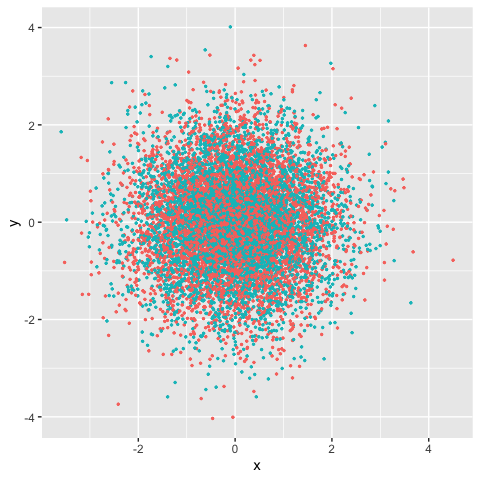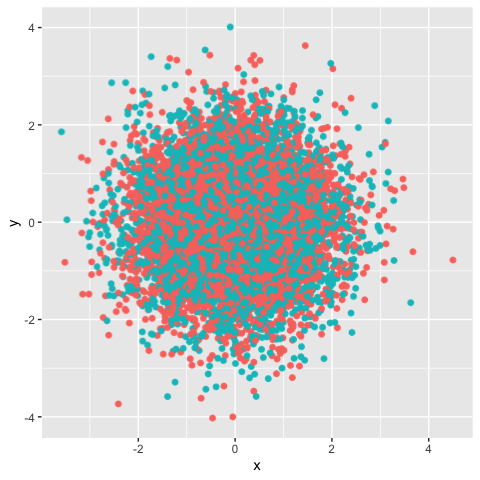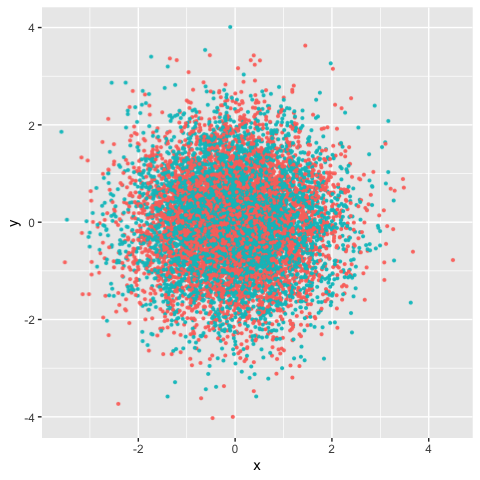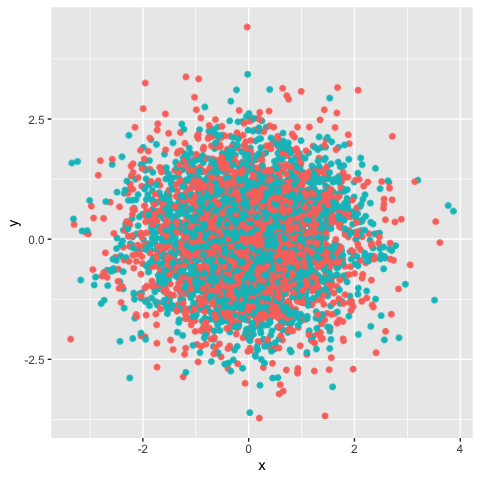# Legacy (outdated) wrapper functions

For historic reasons, `ggrastr` used to be solely composed of the following functions:

• `geom_point_rast`: raster scatter plots
• `geom_jitter_rast`: raster jittered scatter plots
• `geom_boxplot_jitter`: boxplots that allows to jitter and rasterize outlier points
• `geom_tile_rast`: raster heatmap
• `geom_beeswarm_rast`: raster bee swarm plots
• `geom_quasirandom_rast`: raster quasirandom scatter plot

However, we strongly encourage users to use the `rasterise()` function instead. For posterity’s sake, we have only included the old vignettes here for the reference of users, along with the equivalent functions using `rasterise()`.

#### Points: Rasterize scatter plots with geom_point_rast()

Sometimes you need to publish a figure in a vector format:

``````library(ggplot2)
library(ggrastr)
points_num <- 10000
df <- data.frame(x=rnorm(points_num), y=rnorm(points_num), c=as.factor(1:points_num %% 2))
gg <- ggplot(df, aes(x=x, y=y, color=c)) + scale_color_discrete(guide="none")
gg_vec <- gg + geom_point(size=0.5)
print(gg_vec)``````But in other cases, your figure contains thousands of points, e.g. try `points_num <- 500000` in the example above, and you will notice the performance issues—it takes significantly longer to render the plot.

In this case, a reasonable solution would be to rasterize the plot. But the problem is that all text becomes rasterized as well.

Raster layers with `ggrastr` were developed to prevent such a situation, using `rasterized

``````gg_rasterized <- gg + rasterise(geom_point(), dpi = 300, scale = 1)
print(gg_rasterized)``````The legacy function used in older versions of ggrastr was `geom_point_rast()`:

``````gg_rast <- gg + geom_point_rast(size=0.5)
print(gg_rast)``````The plots look the same, but the difference in size can be seen when they are exported to pdfs. Unfortunately, there is a longer rendering time to produce such plots:

``````PrintFileSize <- function(gg, name) {
invisible(ggsave('tmp.pdf', gg, width=4, height=4))
cat(name, ': ', file.info('tmp.pdf')\$size / 1024, ' Kb.\n', sep = '')
}
PrintFileSize(gg_rast, 'Raster')
#> Raster: 291.5576 Kb.
PrintFileSize(gg_vec, 'Vector')
#> Vector: 556.1484 Kb.``````

As expected, the difference becomes larger with growth of number of points:

``````points_num <- 1000000
df <- data.frame(x=rnorm(points_num), y=rnorm(points_num), c=as.factor(1:points_num %% 2))
gg <- ggplot(df, aes(x=x, y=y, color=c)) + scale_color_discrete(guide="none")
gg_vec <- gg + geom_point(size=0.5)
gg_rast <- gg + geom_point_rast(size=0.5)
PrintFileSize(gg_rast, 'Raster')
#> Raster: 358.6611 Kb.
PrintFileSize(gg_vec, 'Vector')
#> Vector: 54786.11 Kb.``````

#### Jitter: Rasterize jittered scatter plots with geom_jitter_rast()

Users may also opt to create rasterized scatter plots with jitter:

``````library(ggplot2)
library(ggrastr)
points_num <- 5000
df <- data.frame(x=rnorm(points_num), y=rnorm(points_num), c=as.factor(1:points_num %% 2))
gg <- ggplot(df, aes(x=x, y=y, color=c)) + scale_color_discrete(guide="none")
gg_jitter_rast <- gg + rasterise(geom_jitter(), dpi = 300, scale = 1)
print(gg_jitter_rast)``````The legacy wrapper `geom_jitter_rast()` used the following syntax:

``````library(ggplot2)
library(ggrastr)

points_num <- 5000
df <- data.frame(x=rnorm(points_num), y=rnorm(points_num), c=as.factor(1:points_num %% 2))
gg <- ggplot(df, aes(x=x, y=y, color=c)) + scale_color_discrete(guide=FALSE)

gg_jitter_rast <- gg + geom_jitter_rast(raster.dpi=600)
print(gg_jitter_rast)
#> Warning: It is deprecated to specify `guide = FALSE` to remove a guide. Please
#> use `guide = "none"` instead.``````